# 1. 贝叶斯景象图

## 0x1：先验分布的可视化图景像

### 1. 二维均匀分布的先验图景像

# -*- coding: utf-8 -*-

import scipy.stats as stats
from IPython.core.pylabtools import figsize
import numpy as np
figsize(12.5, 4)

import matplotlib.pyplot as plt
from mpl_toolkits.mplot3d import Axes3D

jet = plt.cm.jet
fig = plt.figure()
x = y = np.linspace(0, 5, 100)
X, Y = np.meshgrid(x, y)

plt.subplot(121)
uni_x = stats.uniform.pdf(x, loc=0, scale=5)　　# 均匀分布
uni_y = stats.uniform.pdf(y, loc=0, scale=5)　　# 均匀分布
M = np.dot(uni_y[:, None], uni_x[None, :])
im = plt.imshow(M, interpolation='none', origin='lower',
cmap=jet, vmax=1, vmin=-.15, extent=(0, 5, 0, 5))

plt.xlim(0, 5)
plt.ylim(0, 5)
plt.title("Landscape formed by Uniform priors.")

ax.plot_surface(X, Y, M, cmap=plt.cm.jet, vmax=1, vmin=-.15)
ax.view_init(azim=390)
plt.title("Uniform prior landscape; alternate view")plt.show()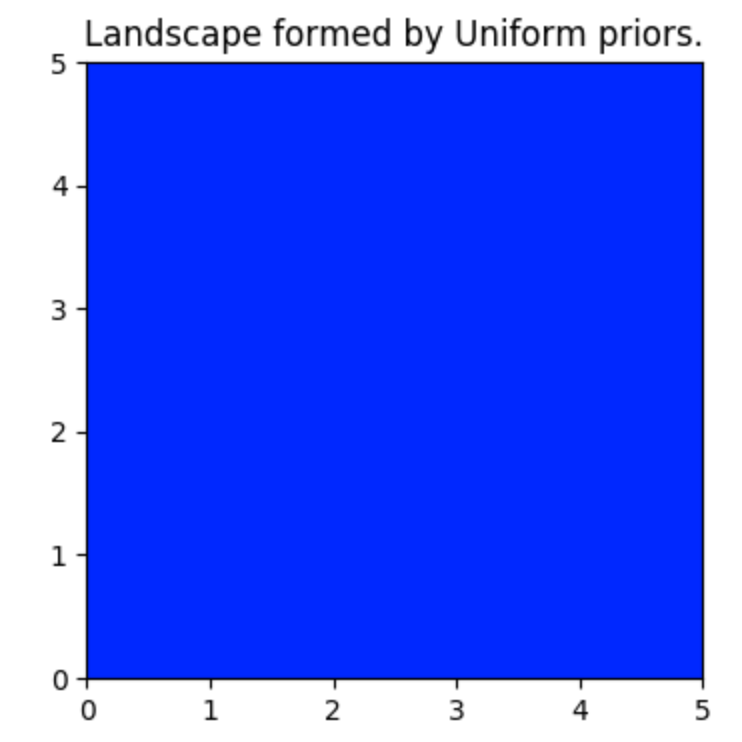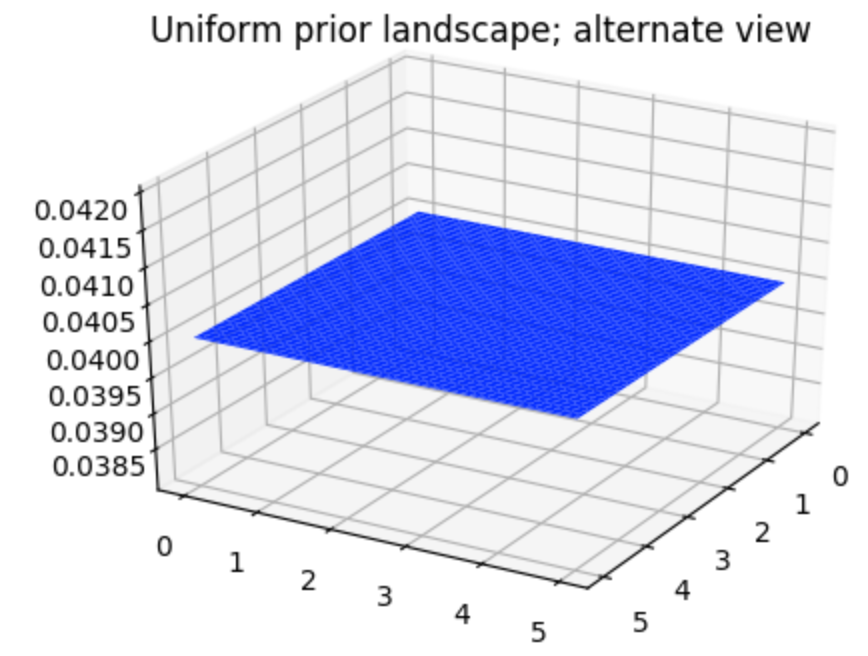### 2. 二维指数分布的先验图景像

# -*- coding: utf-8 -*-

import scipy.stats as stats
from IPython.core.pylabtools import figsize
import numpy as np
figsize(12.5, 4)

import matplotlib.pyplot as plt
from mpl_toolkits.mplot3d import Axes3D

jet = plt.cm.jet
fig = plt.figure()
x = y = np.linspace(0, 5, 100)  # x,y 为[0,5]内的均匀分布
X, Y = np.meshgrid(x, y)

fig = plt.figure()
plt.subplot(121)

exp_x = stats.expon.pdf(x, scale=3)
exp_y = stats.expon.pdf(x, scale=10)
M = np.dot(exp_y[:, None], exp_x[None, :])
CS = plt.contour(X, Y, M)
im = plt.imshow(M, interpolation='none', origin='lower',
cmap=jet, extent=(0, 5, 0, 5))
#plt.xlabel("prior on $p_1$")
#plt.ylabel("prior on $p_2$")
plt.title("$Exp(3), Exp(10)$ prior landscape")

ax.plot_surface(X, Y, M, cmap=jet)
ax.view_init(azim=390)
plt.title("$Exp(3), Exp(10)$ prior landscape; \nalternate view")

plt.show()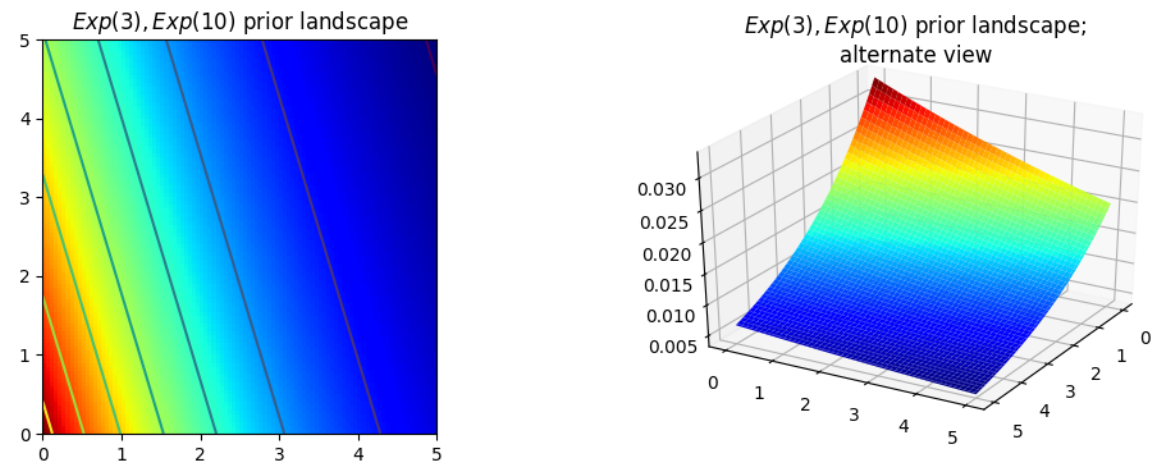## 0x2：观测样本是如何影响未知变量的先验分布的？

### 1. 不同的先验概率对观测样本调整后验分布的阻力是不同的

# -*- coding: utf-8 -*-

import numpy as np
import matplotlib.pyplot as plt
import pymc as pm
from scipy.stats.mstats import mquantiles
from separation_plot import separation_plot
import scipy.stats as stats

jet = plt.cm.jet

# create the observed data

# sample size of data we observe, trying varying this (keep it less than 100 ;)
N = 1

# the true parameters, but of course we do not see these values...
lambda_1_true = 1
lambda_2_true = 3

#...we see the data generated, dependent on the above two values.
data = np.concatenate([
stats.poisson.rvs(lambda_1_true, size=(N, 1)),
stats.poisson.rvs(lambda_2_true, size=(N, 1))
], axis=1)
print("observed (2-dimensional,sample size = %d):" % N, data)

# plotting details.
x = y = np.linspace(.01, 5, 100)
likelihood_x = np.array([stats.poisson.pmf(data[:, 0], _x)
for _x in x]).prod(axis=1)
likelihood_y = np.array([stats.poisson.pmf(data[:, 1], _y)
for _y in y]).prod(axis=1)
L = np.dot(likelihood_x[:, None], likelihood_y[None, :])

# matplotlib heavy lifting below, beware!
plt.subplot(221)
uni_x = stats.uniform.pdf(x, loc=0, scale=5)
uni_y = stats.uniform.pdf(x, loc=0, scale=5)
M = np.dot(uni_y[:, None], uni_x[None, :])
im = plt.imshow(M, interpolation='none', origin='lower',
cmap=jet, vmax=1, vmin=-.15, extent=(0, 5, 0, 5))
plt.scatter(lambda_2_true, lambda_1_true, c="k", s=50, edgecolor="none")
plt.xlim(0, 5)
plt.ylim(0, 5)
plt.title("Landscape formed by Uniform priors on $p_1, p_2$.")

plt.subplot(223)
plt.contour(x, y, M * L)
im = plt.imshow(M * L, interpolation='none', origin='lower',
cmap=jet, extent=(0, 5, 0, 5))
plt.title("Landscape warped by %d data observation;\n Uniform priors on $p_1, p_2$." % N)
plt.scatter(lambda_2_true, lambda_1_true, c="k", s=50, edgecolor="none")
plt.xlim(0, 5)
plt.ylim(0, 5)

plt.subplot(222)
exp_x = stats.expon.pdf(x, loc=0, scale=3)
exp_y = stats.expon.pdf(x, loc=0, scale=10)
M = np.dot(exp_y[:, None], exp_x[None, :])

plt.contour(x, y, M)
im = plt.imshow(M, interpolation='none', origin='lower',
cmap=jet, extent=(0, 5, 0, 5))
plt.scatter(lambda_2_true, lambda_1_true, c="k", s=50, edgecolor="none")
plt.xlim(0, 5)
plt.ylim(0, 5)
plt.title("Landscape formed by Exponential priors on $p_1, p_2$.")

plt.subplot(224)
# This is the likelihood times prior, that results in the posterior.
plt.contour(x, y, M * L)
im = plt.imshow(M * L, interpolation='none', origin='lower',
cmap=jet, extent=(0, 5, 0, 5))

plt.scatter(lambda_2_true, lambda_1_true, c="k", s=50, edgecolor="none")
plt.title("Landscape warped by %d data observation;\n Exponential priors on \
$p_1, p_2$." % N)
plt.xlim(0, 5)
plt.ylim(0, 5)

plt.show()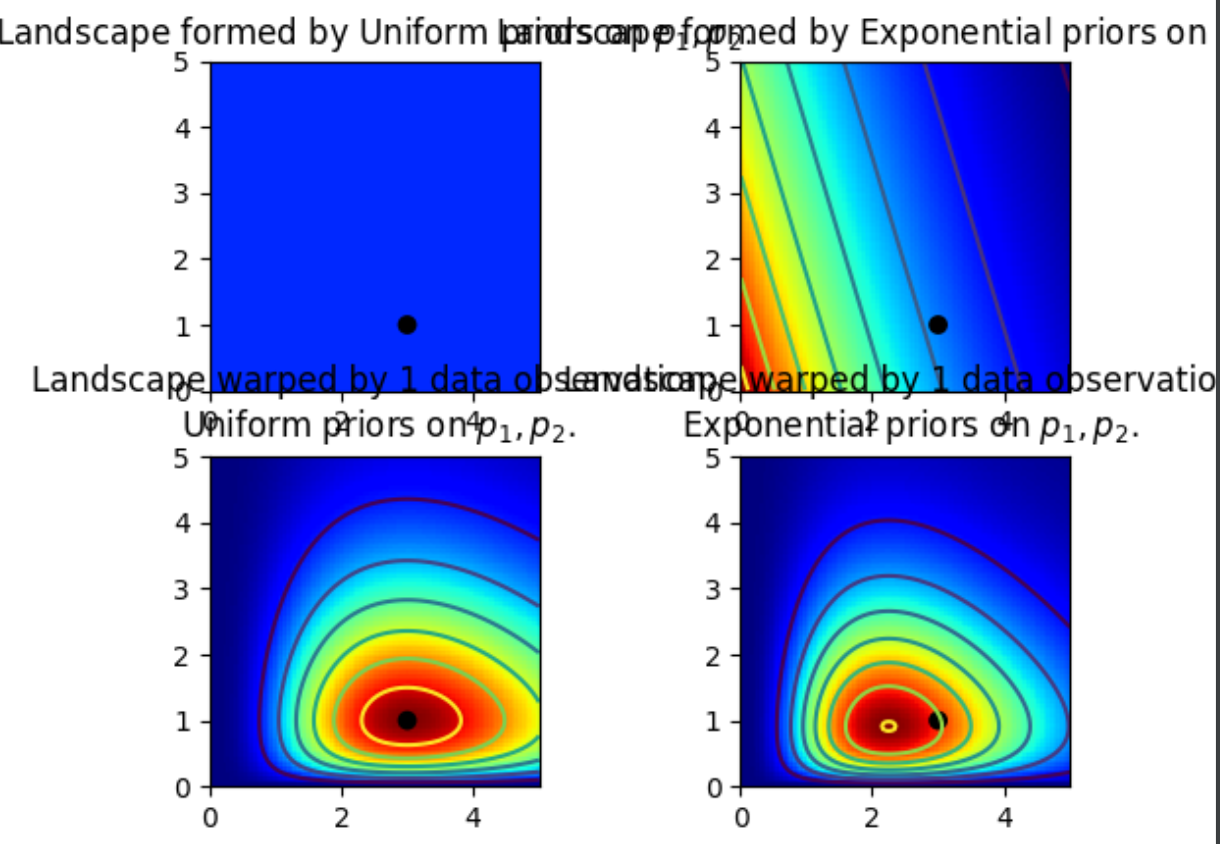1. 右下方的指数先验对应的后验分布图形中，右上角区域的取值很低，原因是假设的指数先验在这一区域的取值也较低。
2. 另一方面，左下方的均匀分布对应的后验分布图形中，右上角区域的取值相对较高，这也是因为均匀先验在该区域的取值相比指数先验更高。3. 在右下角指数分布的后验图形中，最高的山峰，也就是红色最深的地方，向（0，0）点偏斜，原因就是指数先验在这个角落的取值更高。

## 0x3：使用MCMC来搜索图景像

### 1. 为何是上千的样本？

1. 用解析表达式描述“山峰区域”(后验分布)，这需要描述带有山峰和山谷的 N 维曲面。在数学上是比较困难的。
2. 也可以返回图形里的顶峰，这种方法在数学上是可行的，也很好理解（这对应了关于未知量的估计里最可能的取值），但是这种做法忽略了图形的形状，而我们知道，在一些场景下，这些形状对于判定未知变量的后验概率来说，是非常关键的
3. 除了计算原因，另一个主要原因是，利用返回的大量样本可以利用大数定理解决非常棘手问题。有了成千上万的样本，就可以利用直方图技术，重构后验分布曲面。

### 2. MCMC的算法实现

1. 选定初始位置。即选定先验分布的初始位置。
2. 移动到一个新的位置(探索附件的点)。
3. 根据新数据位置和先验分布的紧密程度拒绝或接受新的数据点(石头是否来自于期望的那座山上)。
4.
A. 如果接受，移动到新的点，返回到步骤 1。
B. 否则不移动到新的点返回到步骤 1。
5. 在经过大量的迭代之后，返回所有接受的点。

## 0x4：一个MCMC搜索图景像的实际的例子 - 使用混合模型进行无监督聚类

# -*- coding: utf-8 -*-

import numpy as np
import matplotlib.pyplot as plt
import pymc as pm
import scipy.stats as stats

jet = plt.cm.jet

plt.hist(data, bins=20, color="k", histtype="stepfilled", alpha=0.8)
plt.title("Histogram of the dataset")
plt.ylim([0, None])
print(data[:10], "...")

fig = plt.figure()
ax.hist(data[:], bins=20)

plt.show()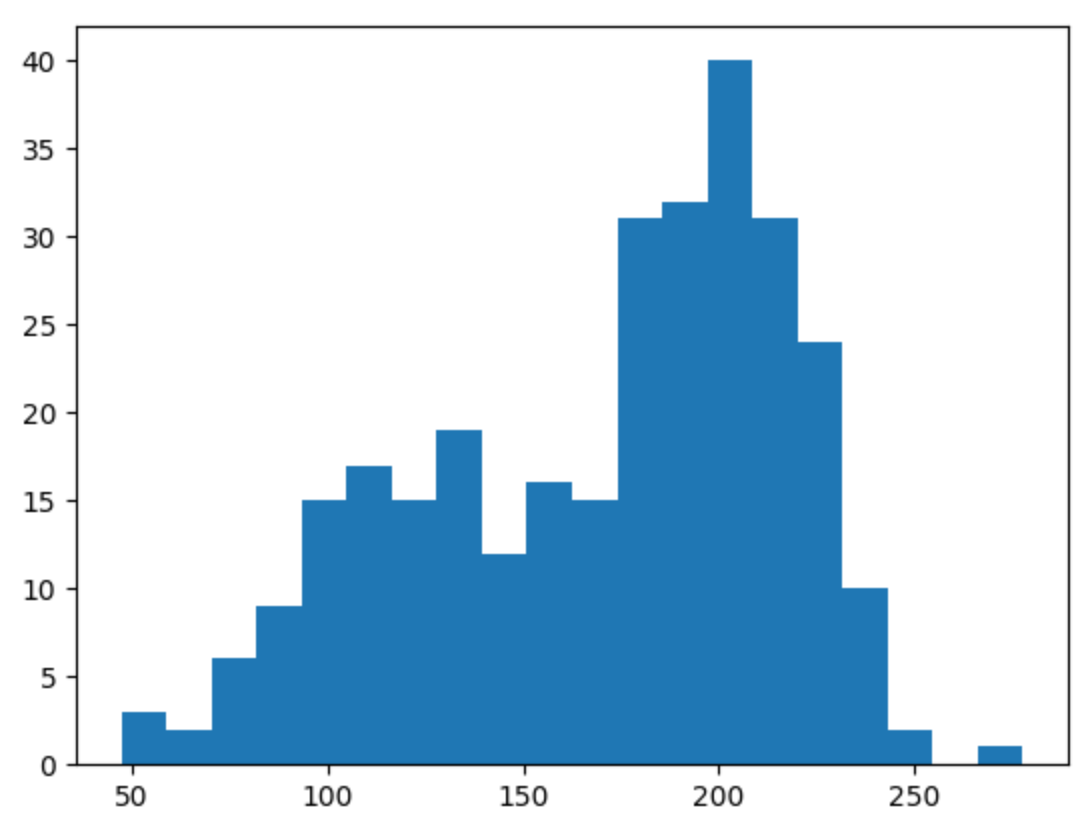### 1. 选择符合数据观测分布的数学模型

1. 对于每个数据点，让它以概率𝑝属于类别 1，以概率1-𝑝属于类别 2。
2. 画出均值 𝜇𝑖 和方差 𝜎𝑖 的正态分布的随机变量，𝑖是 1 中的类别 id，可以取 1 或者 2。
3. 重复 1，2 步骤。 

### 2. 对模型的参数进行先验建模

#### 1）所属类别分布先验

import pymc as pm
p = pm.Uniform("p", 0, 1)
assignment = pm.Categorical("assignment", [p, 1 - p], size=data.shape) print "prior assignment, with p = %.2f:" % p.value
print assignment.value[:10], "..."

#### 2）单个聚类中的正态分布的参数分布先验

 taus = 1.0 / pm.Uniform("stds", 0, 100, size=2) ** 2
centers = pm.Normal("centers", [120, 190], [0.01, 0.01], size=2)
"""
The below deterministic functions map an assignment, in this case 0 or 1, to a set of parameters, located in the (1,2) arrays taus and centers. """
@pm.deterministic
def center_i(assignment=assignment, centers=centers):
return centers[assignment] @pm.deterministic
def tau_i(assignment=assignment, taus=taus):
return taus[assignment]
print "Random assignments: ", assignment.value[:4], "..." print "Assigned center: ", center_i.value[:4], "..."
print "Assigned precision: ", tau_i.value[:4], "..."

### 3. MCMC搜索过程 - 迹

PyMC 有个 MCMC 类，MCMC 位于 PyMC 名称空间中，其实现了 MCMC 算法。用 Model 对象实例化 MCMC 类，如下

mcmc = pm.MCMC( model )

mcmc = pm.MCMC(model) mcmc.sample(50000)

mcmc.trace("centers")

# -*- coding: utf-8 -*-

import numpy as np
import matplotlib.pyplot as plt
import pymc as pm
import scipy.stats as stats

jet = plt.cm.jet

p = pm.Uniform("p", 0, 1)

assignment = pm.Categorical("assignment", [p, 1 - p], size=data.shape)
print("prior assignment, with p = %.2f:" % p.value)
print(assignment.value[:10], "...")

stds = pm.Uniform("stds", 0, 100, size=2)
taus = 1.0 / stds ** 2
centers = pm.Normal("centers", [120, 190], [0.01, 0.01], size=2)

"""
The below deterministic functions map an assignment, in this case 0 or 1,
to a set of parameters, located in the (1,2) arrays taus and centers.
"""

@pm.deterministic
def center_i(assignment=assignment, centers=centers):
return centers[assignment]

@pm.deterministic
def tau_i(assignment=assignment, taus=taus):
return taus[assignment]

print("Random assignments: ", assignment.value[:4], "...")
print("Assigned center: ", center_i.value[:4], "...")
print("Assigned precision: ", tau_i.value[:4], "...")

# and to combine it with the observations:
observations = pm.Normal("obs", center_i, tau_i, value=data, observed=True)

# below we create a model class
model = pm.Model([p, assignment, observations, taus, centers])

mcmc = pm.MCMC(model)
mcmc.sample(50000)

plt.subplot(311)
lw = 1
center_trace = mcmc.trace("centers")[:]

# for pretty colors later in the book.
colors = ["#348ABD", "#A60628"] \
if center_trace[-1, 0] > center_trace[-1, 1] \
else ["#A60628", "#348ABD"]

plt.plot(center_trace[:, 0], label="trace of center 0", c=colors, lw=lw)
plt.plot(center_trace[:, 1], label="trace of center 1", c=colors, lw=lw)
plt.title("Traces of unknown parameters")
leg = plt.legend(loc="upper right")
leg.get_frame().set_alpha(0.7)

plt.subplot(312)
std_trace = mcmc.trace("stds")[:]
plt.plot(std_trace[:, 0], label="trace of standard deviation of cluster 0",
c=colors, lw=lw)
plt.plot(std_trace[:, 1], label="trace of standard deviation of cluster 1",
c=colors, lw=lw)
plt.legend(loc="upper left")

plt.subplot(313)
p_trace = mcmc.trace("p")[:]
plt.plot(p_trace, label="$p$: frequency of assignment to cluster 0",
color="#467821", lw=lw)
plt.xlabel("Steps")
plt.ylim(0, 1)
plt.legend()

plt.show()1. 这些迹并非收敛到某一点，而是收敛到一定分布下，概率较大的点集。这就是MCMC算法里收敛的涵义。
2. 最初的几千个点（训练轮）与最终的目标分布关系不大，所以使用这些点参与估计并不明智。一个聪明的做法是剔除这些点之后再执行估计，产生这些遗弃点的过程称为预热期。
3. 这些迹看起来像是在围绕空间中某一区域随机游走。这就是说它总是在基于之前的位置移动。这样的好处是确保了当前位置与之前位置之间存在直接、确定的联系。然而坏处就是太过于限制探索空间的效率。

### 4. 如何估计各个未知变量的最佳后验估计值

# -*- coding: utf-8 -*-

import numpy as np
import matplotlib.pyplot as plt
import pymc as pm
import scipy.stats as stats

jet = plt.cm.jet

p = pm.Uniform("p", 0, 1)

assignment = pm.Categorical("assignment", [p, 1 - p], size=data.shape)
print("prior assignment, with p = %.2f:" % p.value)
print(assignment.value[:10], "...")

stds = pm.Uniform("stds", 0, 100, size=2)
taus = 1.0 / stds ** 2
centers = pm.Normal("centers", [120, 190], [0.01, 0.01], size=2)

"""
The below deterministic functions map an assignment, in this case 0 or 1,
to a set of parameters, located in the (1,2) arrays taus and centers.
"""

@pm.deterministic
def center_i(assignment=assignment, centers=centers):
return centers[assignment]

@pm.deterministic
def tau_i(assignment=assignment, taus=taus):
return taus[assignment]

print("Random assignments: ", assignment.value[:4], "...")
print("Assigned center: ", center_i.value[:4], "...")
print("Assigned precision: ", tau_i.value[:4], "...")

# and to combine it with the observations:
observations = pm.Normal("obs", center_i, tau_i, value=data, observed=True)

# below we create a model class
model = pm.Model([p, assignment, observations, taus, centers])

mcmc = pm.MCMC(model)
mcmc.sample(50000)

# 获取整条迹
std_trace = mcmc.trace("stds")[:]
center_trace = mcmc.trace("centers")[:]

# for pretty colors later in the book.
colors = ["#348ABD", "#A60628"] \
if center_trace[-1, 0] > center_trace[-1, 1] \
else ["#A60628", "#348ABD"]

_i = [1, 2, 3, 4]
for i in range(2):
plt.subplot(2, 2, _i[2 * i])
plt.title("Posterior of center of cluster %d" % i)
plt.hist(center_trace[:, i], color=colors[i], bins=30,
histtype="stepfilled")

plt.subplot(2, 2, _i[2 * i + 1])
plt.title("Posterior of standard deviation of cluster %d" % i)
plt.hist(std_trace[:, i], color=colors[i], bins=30,
histtype="stepfilled")
# plt.autoscale(tight=True)

plt.tight_layout()

plt.show()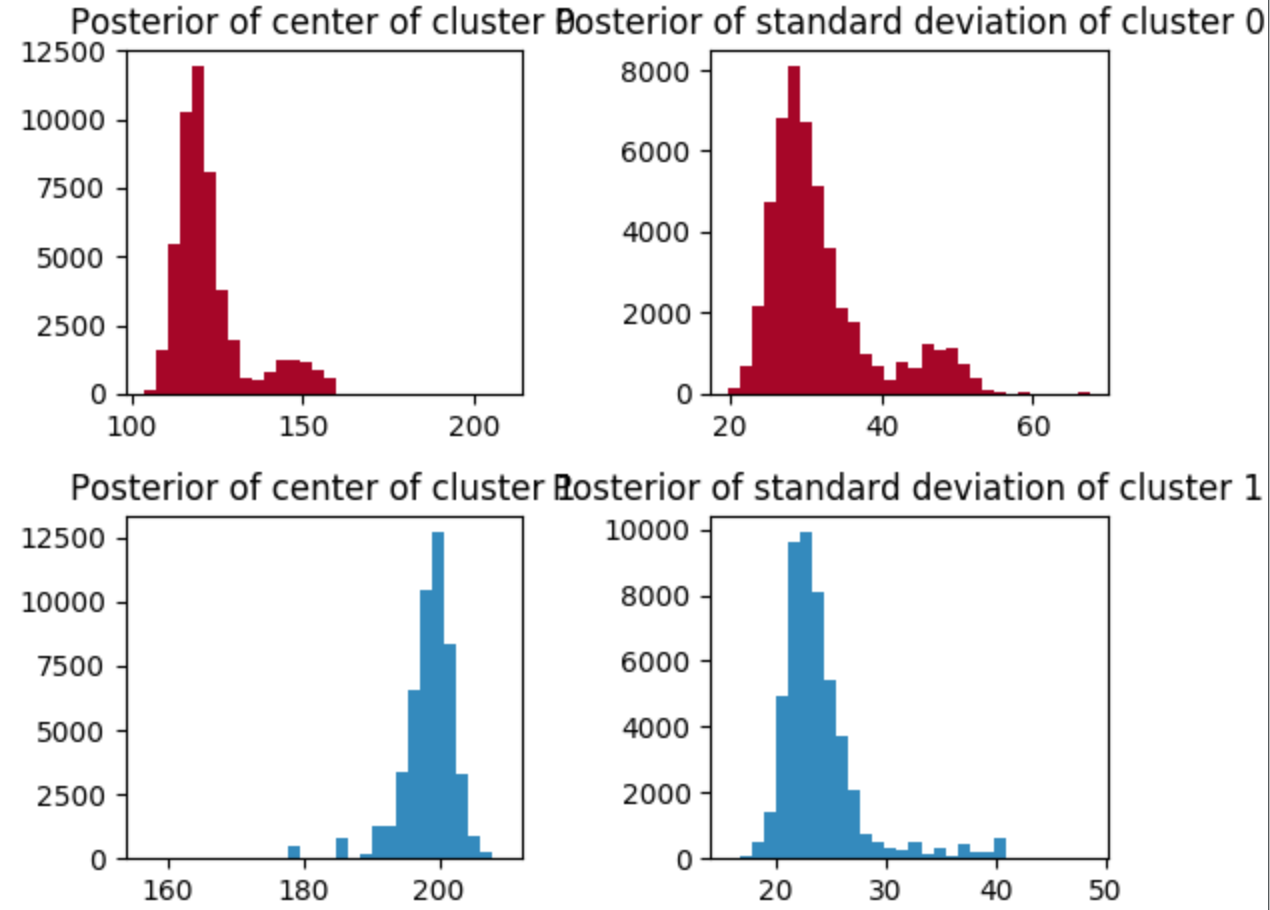# -*- coding: utf-8 -*-

import numpy as np
import matplotlib.pyplot as plt
import pymc as pm
import scipy.stats as stats
import matplotlib as mpl

jet = plt.cm.jet

p = pm.Uniform("p", 0, 1)

assignment = pm.Categorical("assignment", [p, 1 - p], size=data.shape)
print("prior assignment, with p = %.2f:" % p.value)
print(assignment.value[:10], "...")

stds = pm.Uniform("stds", 0, 100, size=2)
taus = 1.0 / stds ** 2
centers = pm.Normal("centers", [120, 190], [0.01, 0.01], size=2)

"""
The below deterministic functions map an assignment, in this case 0 or 1,
to a set of parameters, located in the (1,2) arrays taus and centers.
"""

@pm.deterministic
def center_i(assignment=assignment, centers=centers):
return centers[assignment]

@pm.deterministic
def tau_i(assignment=assignment, taus=taus):
return taus[assignment]

print("Random assignments: ", assignment.value[:4], "...")
print("Assigned center: ", center_i.value[:4], "...")
print("Assigned precision: ", tau_i.value[:4], "...")

# and to combine it with the observations:
observations = pm.Normal("obs", center_i, tau_i, value=data, observed=True)

# below we create a model class
model = pm.Model([p, assignment, observations, taus, centers])

mcmc = pm.MCMC(model)
mcmc.sample(50000)

center_trace = mcmc.trace("centers")[:]
# for pretty colors later in the book.
colors = ["#348ABD", "#A60628"] \
if center_trace[-1, 0] > center_trace[-1, 1] \
else ["#A60628", "#348ABD"]

plt.cmap = mpl.colors.ListedColormap(colors)
plt.imshow(mcmc.trace("assignment")[::400, np.argsort(data)],
cmap=plt.cmap, aspect=.4, alpha=.9)
plt.xticks(np.arange(0, data.shape, 40),
["%.2f" % s for s in np.sort(data)[::40]])
plt.ylabel("posterior sample")
plt.xlabel("value of $i$th data point")
plt.title("Posterior labels of data points")

plt.show()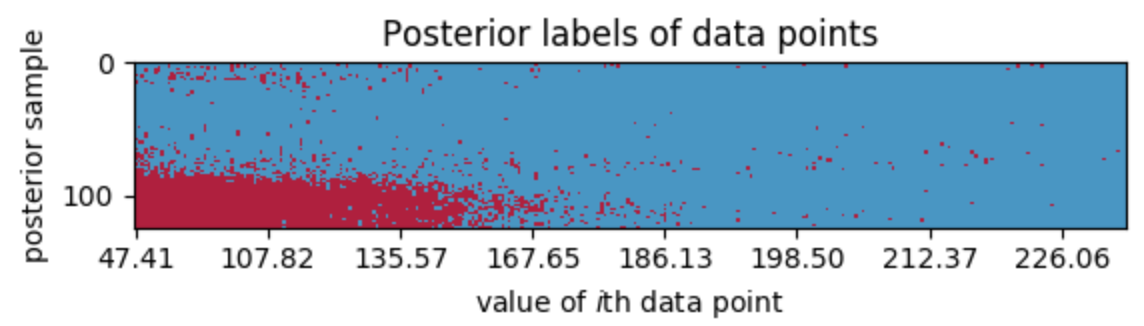x 轴表示先验数据的排序值。 红色的地方表示类别 1，蓝色地方代表类别 0。

# -*- coding: utf-8 -*-

import numpy as np
import matplotlib.pyplot as plt
import pymc as pm
import scipy.stats as stats
import matplotlib as mpl

jet = plt.cm.jet

p = pm.Uniform("p", 0, 1)

assignment = pm.Categorical("assignment", [p, 1 - p], size=data.shape)
print("prior assignment, with p = %.2f:" % p.value)
print(assignment.value[:10], "...")

stds = pm.Uniform("stds", 0, 100, size=2)
taus = 1.0 / stds ** 2
centers = pm.Normal("centers", [120, 190], [0.01, 0.01], size=2)

"""
The below deterministic functions map an assignment, in this case 0 or 1,
to a set of parameters, located in the (1,2) arrays taus and centers.
"""

@pm.deterministic
def center_i(assignment=assignment, centers=centers):
return centers[assignment]

@pm.deterministic
def tau_i(assignment=assignment, taus=taus):
return taus[assignment]

print("Random assignments: ", assignment.value[:4], "...")
print("Assigned center: ", center_i.value[:4], "...")
print("Assigned precision: ", tau_i.value[:4], "...")

# and to combine it with the observations:
observations = pm.Normal("obs", center_i, tau_i, value=data, observed=True)

# below we create a model class
model = pm.Model([p, assignment, observations, taus, centers])

mcmc = pm.MCMC(model)
mcmc.sample(50000)

center_trace = mcmc.trace("centers")[:]
# for pretty colors later in the book.
colors = ["#348ABD", "#A60628"] \
if center_trace[-1, 0] > center_trace[-1, 1] \
else ["#A60628", "#348ABD"]

cmap = mpl.colors.LinearSegmentedColormap.from_list("BMH", colors)
assign_trace = mcmc.trace("assignment")[:]
plt.scatter(data, 1 - assign_trace.mean(axis=0), cmap=cmap,
c=assign_trace.mean(axis=0), s=50)
plt.ylim(-0.05, 1.05)
plt.xlim(35, 300)
plt.title("Probability of data point belonging to cluster 0")
plt.ylabel("probability")
plt.xlabel("value of data point")

plt.show()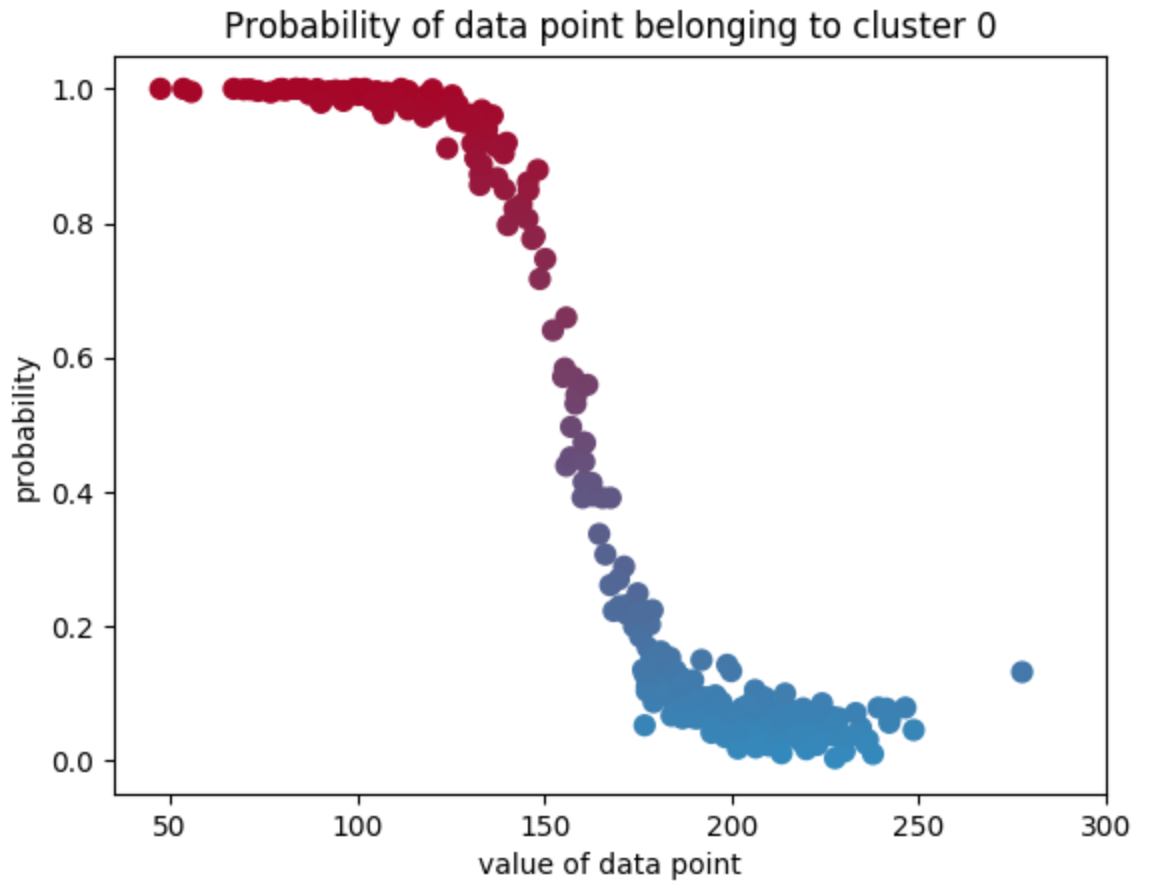# -*- coding: utf-8 -*-

import numpy as np
import matplotlib.pyplot as plt
import pymc as pm
import scipy.stats as stats
import matplotlib as mpl

jet = plt.cm.jet

p = pm.Uniform("p", 0, 1)

assignment = pm.Categorical("assignment", [p, 1 - p], size=data.shape)
print("prior assignment, with p = %.2f:" % p.value)
print(assignment.value[:10], "...")

stds = pm.Uniform("stds", 0, 100, size=2)
taus = 1.0 / stds ** 2
centers = pm.Normal("centers", [120, 190], [0.01, 0.01], size=2)

"""
The below deterministic functions map an assignment, in this case 0 or 1,
to a set of parameters, located in the (1,2) arrays taus and centers.
"""

@pm.deterministic
def center_i(assignment=assignment, centers=centers):
return centers[assignment]

@pm.deterministic
def tau_i(assignment=assignment, taus=taus):
return taus[assignment]

print("Random assignments: ", assignment.value[:4], "...")
print("Assigned center: ", center_i.value[:4], "...")
print("Assigned precision: ", tau_i.value[:4], "...")

# and to combine it with the observations:
observations = pm.Normal("obs", center_i, tau_i, value=data, observed=True)

# below we create a model class
model = pm.Model([p, assignment, observations, taus, centers])

mcmc = pm.MCMC(model)
mcmc.sample(50000)

center_trace = mcmc.trace("centers")[:]
# for pretty colors later in the book.
colors = ["#348ABD", "#A60628"] \
if center_trace[-1, 0] > center_trace[-1, 1] \
else ["#A60628", "#348ABD"]

std_trace = mcmc.trace("stds")[:]

norm = stats.norm
x = np.linspace(20, 300, 500)
posterior_center_means = center_trace.mean(axis=0)
posterior_std_means = std_trace.mean(axis=0)
posterior_p_mean = mcmc.trace("p")[:].mean()

plt.hist(data, bins=20, histtype="step", normed=True, color="k",
lw=2, label="histogram of data")
y = posterior_p_mean * norm.pdf(x, loc=posterior_center_means,
scale=posterior_std_means)
plt.plot(x, y, label="Cluster 0 (using posterior-mean parameters)", lw=3)
plt.fill_between(x, y, color=colors, alpha=0.3)

y = (1 - posterior_p_mean) * norm.pdf(x, loc=posterior_center_means,
scale=posterior_std_means)
plt.plot(x, y, label="Cluster 1 (using posterior-mean parameters)", lw=3)
plt.fill_between(x, y, color=colors, alpha=0.3)

plt.legend(loc="upper left")
plt.title("Visualizing Clusters using posterior-mean parameters")

plt.show()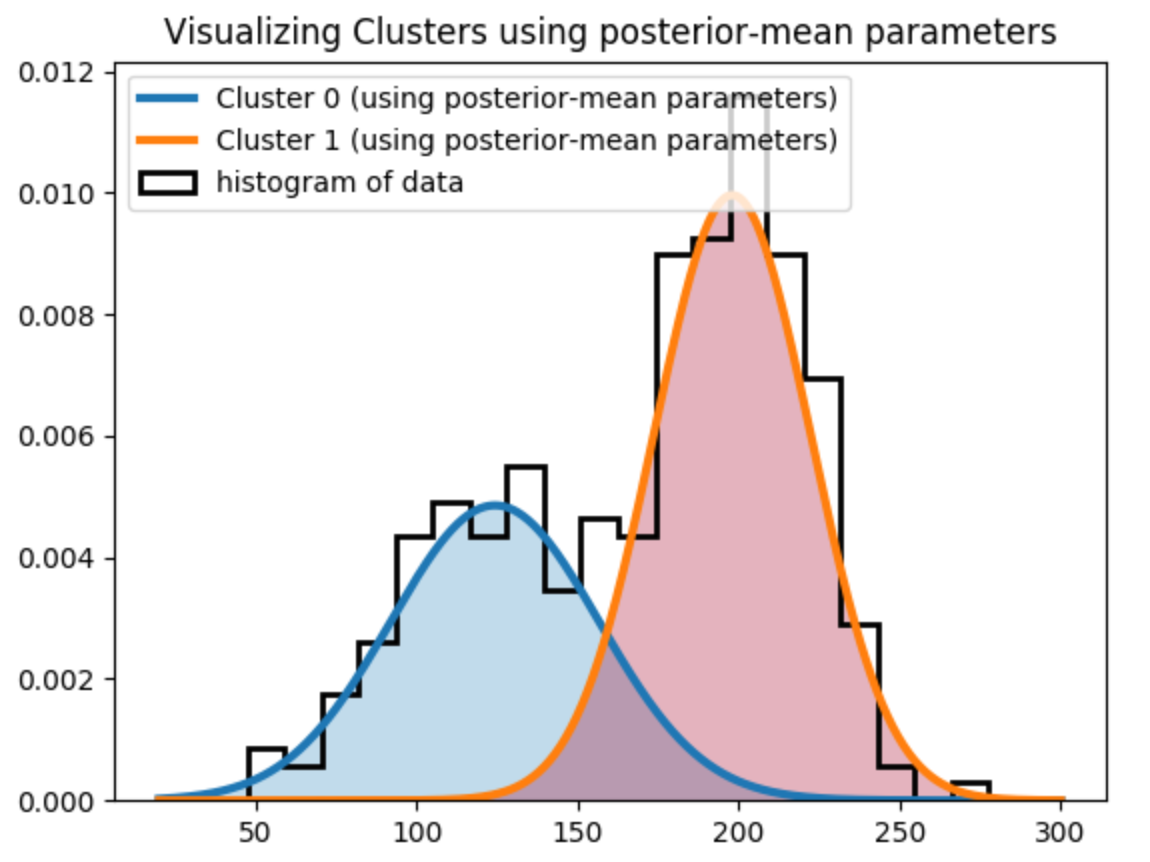### 5. 回到聚类：预测问题 - 到了该决策的时候了！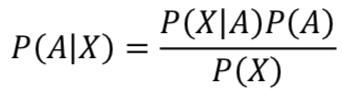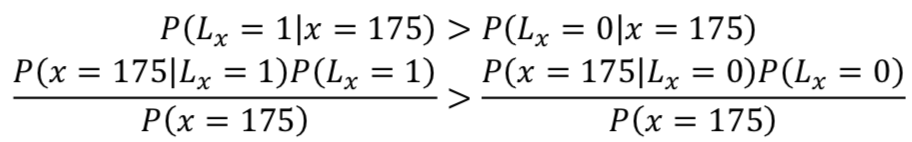# -*- coding: utf-8 -*-

import numpy as np
import matplotlib.pyplot as plt
import pymc as pm
import scipy.stats as stats
import matplotlib as mpl

jet = plt.cm.jet

p = pm.Uniform("p", 0, 1)

assignment = pm.Categorical("assignment", [p, 1 - p], size=data.shape)
print("prior assignment, with p = %.2f:" % p.value)
print(assignment.value[:10], "...")

stds = pm.Uniform("stds", 0, 100, size=2)
taus = 1.0 / stds ** 2
centers = pm.Normal("centers", [120, 190], [0.01, 0.01], size=2)

"""
The below deterministic functions map an assignment, in this case 0 or 1,
to a set of parameters, located in the (1,2) arrays taus and centers.
"""

@pm.deterministic
def center_i(assignment=assignment, centers=centers):
return centers[assignment]

@pm.deterministic
def tau_i(assignment=assignment, taus=taus):
return taus[assignment]

print("Random assignments: ", assignment.value[:4], "...")
print("Assigned center: ", center_i.value[:4], "...")
print("Assigned precision: ", tau_i.value[:4], "...")

# and to combine it with the observations:
observations = pm.Normal("obs", center_i, tau_i, value=data, observed=True)

# below we create a model class
model = pm.Model([p, assignment, observations, taus, centers])

mcmc = pm.MCMC(model)
mcmc.sample(50000)

center_trace = mcmc.trace("centers")[:]
# for pretty colors later in the book.
colors = ["#348ABD", "#A60628"] \
if center_trace[-1, 0] > center_trace[-1, 1] \
else ["#A60628", "#348ABD"]

std_trace = mcmc.trace("stds")[:]

norm_pdf = stats.norm.pdf
p_trace = mcmc.trace("p")[:]
x = 175

v = p_trace * norm_pdf(x, loc=center_trace[:, 0], scale=std_trace[:, 0]) > \
(1 - p_trace) * norm_pdf(x, loc=center_trace[:, 1], scale=std_trace[:, 1])

print("Probability of belonging to cluster 1:", v.mean())


('Probability of belonging to cluster 1:', 0.06006)

# 2. MCMC收敛性讨论

## 0x1：使用MAP来改进收敛性

map_ = pm.MAP( model ) map_.fit()

## 0x2：如何判断收敛？

### 1. 自相关 - 序列递归推演性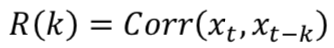𝑥𝑡~Normal(0,1)，𝑥0 =1
𝑦𝑡~Normal(𝑦𝑡−1,1)，𝑦0 = 1

# -*- coding: utf-8 -*-

import numpy as np
import matplotlib.pyplot as plt
import pymc as pm
import scipy.stats as stats
import matplotlib as mpl

x_t = pm.rnormal(0, 1, 200)
x_t = 0
y_t = np.zeros(200)
for i in range(1, 200):
y_t[i] = pm.rnormal(y_t[i - 1], 1)

plt.plot(y_t, label="$y_t$", lw=3)
plt.plot(x_t, label="$x_t$", lw=3)
plt.xlabel("time, $t$")
plt.legend()

plt.show()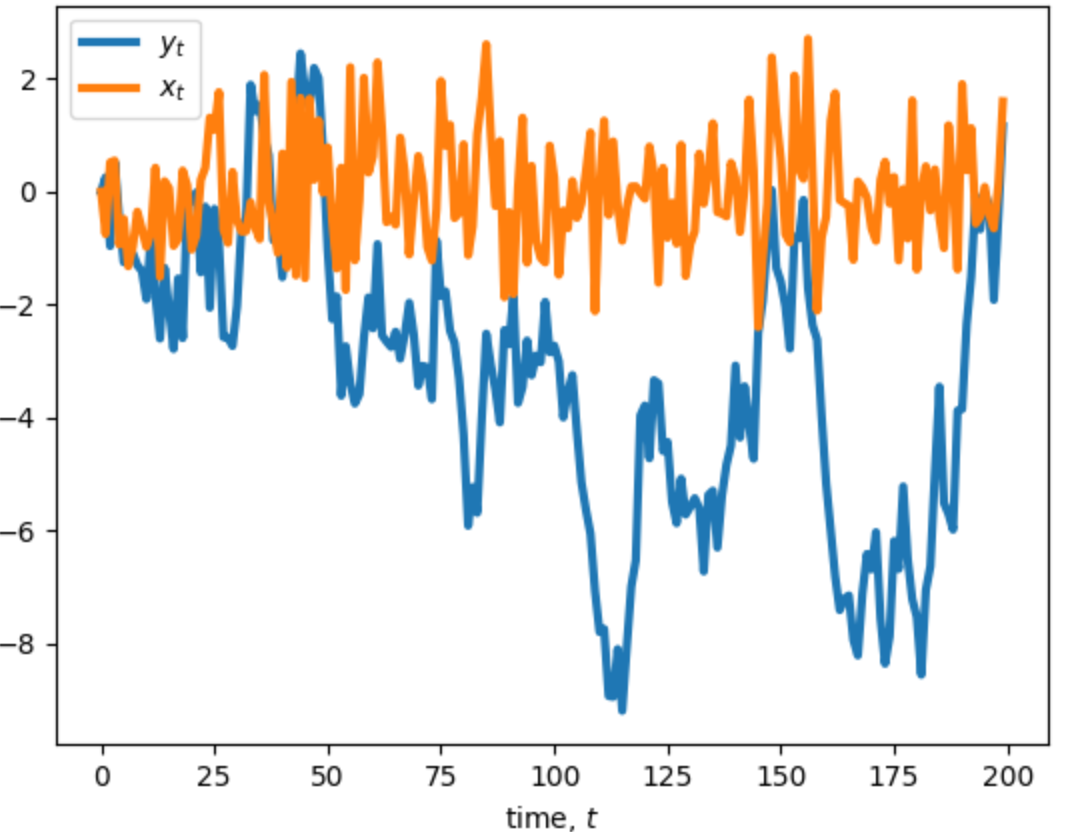# -*- coding: utf-8 -*-

import numpy as np
import matplotlib.pyplot as plt
import pymc as pm
import scipy.stats as stats
import matplotlib as mpl

x_t = pm.rnormal(0, 1, 200)
x_t = 0
y_t = np.zeros(200)
for i in range(1, 200):
y_t[i] = pm.rnormal(y_t[i - 1], 1)

def autocorr(x):
# from http://tinyurl.com/afz57c4
result = np.correlate(x, x, mode='full')
result = result / np.max(result)
return result[result.size // 2:]

colors = ["#348ABD", "#A60628", "#7A68A6"]

x = np.arange(1, 200)
plt.bar(x, autocorr(y_t)[1:], width=1, label="$y_t$",
edgecolor=colors, color=colors)
plt.bar(x, autocorr(x_t)[1:], width=1, label="$x_t$",
color=colors, edgecolor=colors)

plt.legend(title="Autocorrelation")
plt.ylabel("measured correlation \nbetween $y_t$ and $y_{t-k}$.")
plt.xlabel("k (lag)")
plt.title("Autocorrelation plot of $y_t$ and $x_t$ for differing $k$ lags.")

plt.show()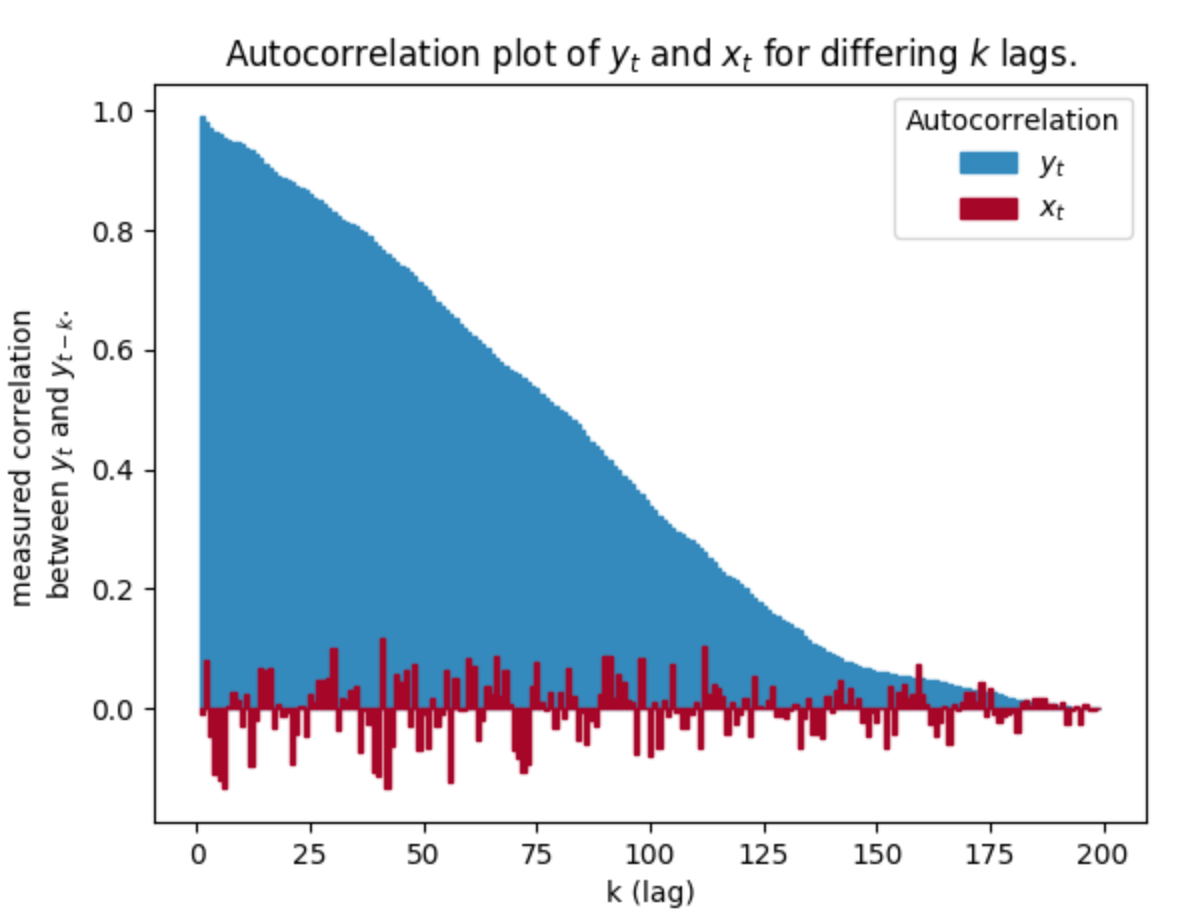### 2. 自相关性和MCM的收敛有何关系？

MCMC算法会天然地返回具有自相关性的采样结果，这是因为MCMC的“摸索性行走”算法：总是从当前位置，移动到附近的某个位置。

# 3. MCMC的一些小技巧

## 0x1：聪明的初始值

mu = pm.Uniform( "mu", 0, 100, value = data.mean() )

## 0x3：统计计算的无名定理

posted @ 2018-08-31 20:58  郑瀚Andrew.Hann  阅读(...)  评论(...编辑  收藏